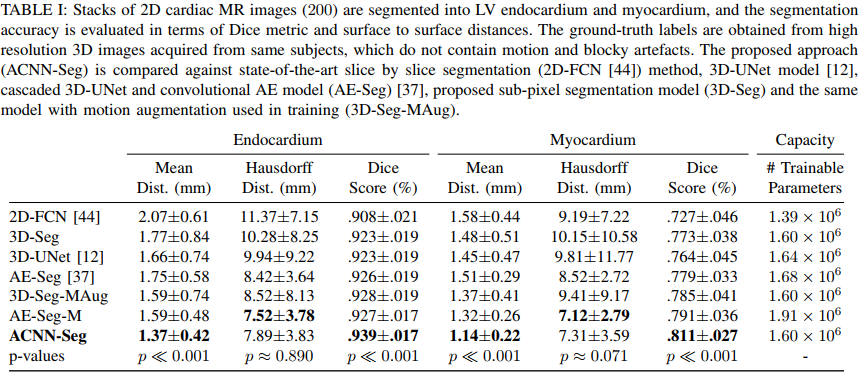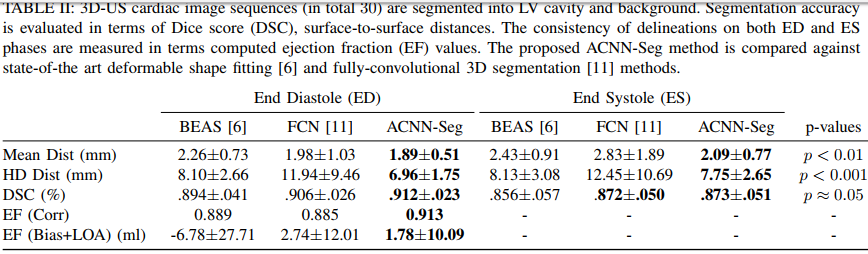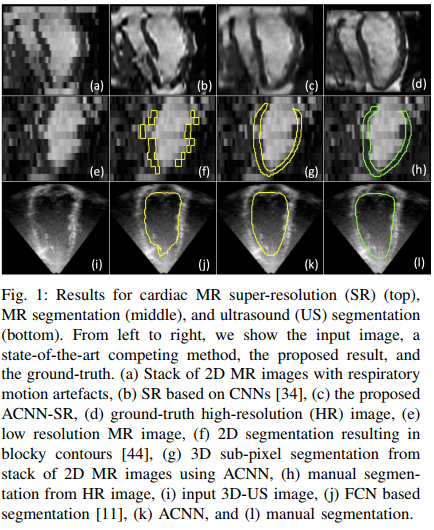## Introduction

They propose a generic and novel technique to incorporate priors on shape and label structure into NNs for cardiac image analysis tasks. For this, they constrain the training process and guide the NN to make anatomically more meaningful predictions. For this, they use a convolutional autoencoder network to learn anatomical shape variations from medical images.

They show that their method works both on MRI and Ultrasound images and can be used for segmenation and super resolution purposes.

## Method

The method is shown in Fig.4. $$\phi$$ is typically a UNet, $$p(.)$$ and $$f(.)$$ are two encoders. As shown in Fig.3, $$p(.)$$ tries to recover the latent vector $$\vec h$$ obtained by the label field autoencoder $$f(.)->g(.)$$.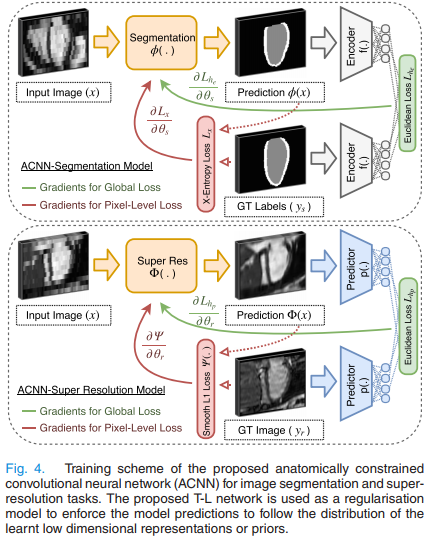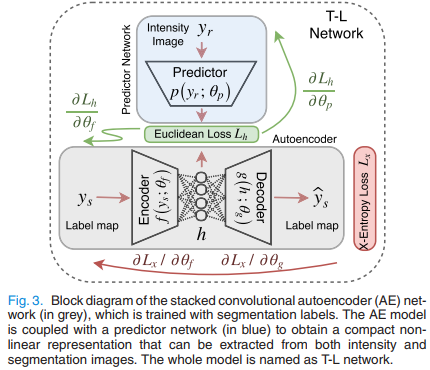The overall segmentation loss to be minimized is: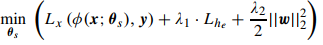where $$x$$ is the input image, $$y$$ the label field, $$f(y)$$ is the encoder for $$y$$, $$L_x(...)$$ is the usual cross-entropy and $$L_{he}$$ is the l2 distance between autoencoder latent vectors: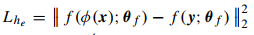The superresolution loss is quite the same but with a L2 likelihood.

## Results

They get state-of-the art results both on 3D echocardiographic images and MR images. They however do not report results on the Right Ventricle and do not report the percentage of anatomically implausible results.